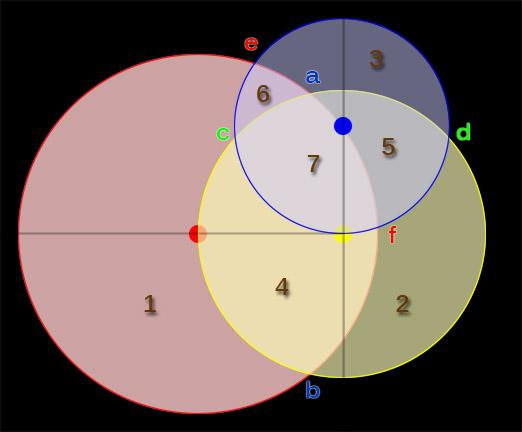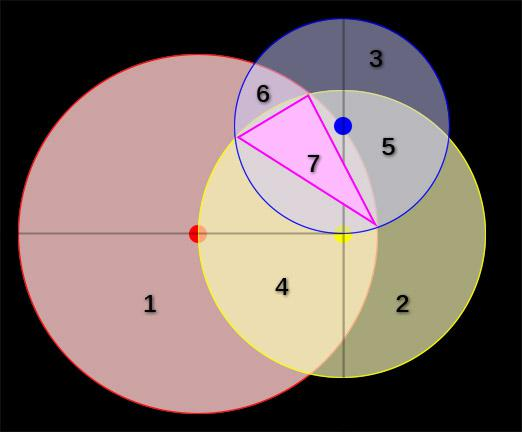BrainDen.com - Brain Teasers
• 0

# Red, yellow, and blue pigment

## Question

I am painting with red, yellow, and blue paint.  All three colors will form 3 circles.  When I used the red, i drew a red circle (disc maybe more appropriate as the circles were filled in with their colors) likewise for yellow and blue.  The red circle had a diameter of 10cm, while the yellow and blue had 8cm and 6cm respectively.  The circles were arranged thus:

1. The blue circle's ring touches the center of yellow

2. The yellow circle's ring touches the center of red

3. The red circle's touches the center of blue.

For the sake of argument let's assume that red + blue + yellow = magenta.  In terms of the painted area...

what percentage of the shape is magenta?

How many colors are represented in the painting?

## Recommended Posts

• 0

Let's finish this puzzle.

We have three circles, overlapping so as to create seven regions with differing overlap. Label these regions 1-7 as shown in the diagram. The circles comprise the following regions:

```Circle 1 (Area=25pi): regions 1 4 6 7
Circle 2 (Area=16pi): regions 2 5 4 7
Circle 3 (Area= 9pi): regions 3 6 5 7```Where circle a and circle b overlap, the area of the overlap can be calculated by standard formulas, given the radii (ra, rb) and center-center distances (d) as follows

```Circles  ra  rb  d  (regions)  area of overlap
1&2       5   4  4   (4 7)      28.383
2&3       4   3  3   (5 7)      17.648
3&1       3   5  5   (6 7)      12.352
Total                           58.352```

Finally, we have already calculated the area of region 7 in a previous post to be region 7 = 9.915. Since the puzzle asks the percentage that region 7 consumes of the total area, we need to calculate the sum of the areas of the seven regions. Adding the areas of the three circles gives 50pi = area of regions [ 1, 2, 3, 2(4), 2(5), 2(6), 3(7) ]. From this we must subtract the areas of overlap: 58.352 = area of regions [ 4, 5, 6, 3(7) ]. Finally we must put back in the area of region 7: 9.915 = area of region [ 7 ].

Total area = 50pi - 58.352 + 9.915 = 108.642, of which region 7 consumes 9.126%

##### Share on other sites

• 1

OK so it's not that hard. There are two parts: (1) Magenta area and (2) total area.

Magenta area

Find the three intersections (vertices) that define the magenta area, then calculate four areas: (1) the triangle having those vertices and (2)-(4) the segment areas (defined by the chords and arcs) that attach respectively to the sides of the triangle. Referring to the diagram, where the triangle has been colored in, so that the segments can be more easily seen, calling the circles 1, 2 and 3 that respectively have radii of 5, 4 and 3, and centering circle 1 at the origin, we can calculate

1. x12 = 3.125; y12 = 3.903
2. x23 = 1.019; y23 = 2.667
3. x13 = 4.997; y13 = 0.171The chord lengths and their associated radii are thus

1. c1 = 4.175; r1 = 5
2. c2 = 2.243; r2 = 4
3. c3 = 4.697; r3 = 3

Heron's formula for the triangle and the standard formula for circular segment area give

• T = 4.682
• s1 = 1.283
• s2 = 0.241
• s3 = 3.708

So the total magenta area is 9.915

Total area

There are three "lens" shapes whose area can be calculated with standard formulas. Left as an exercise for another Denizen:

1. lens12 = areas 4 and 7
2. lens23 = areas 5 and 7
3. lens31 = areas 6 and 7

Total area = pi (25 + 16+ 9) - (lens12 + lens23 + lens 31) + 2(9.915)

Percent of magenta = 100 * 9.915 / Total area.

•1
##### Share on other sites

• 1

Can you explain what the 7th shape is meant to represent?

There aren't seven shapes, only four: three circles and a triangle. Three circles define seven regions. Region 7 is the area common to three circles - the region you call magenta. I broke region 7 into four pieces, each of whose area I could calculate.I did this by joining its vertices with straight lines to form a triangle and three circular segments.Perhaps it's confusing that the label "7" ended up inside the triangle. It does refer to the entire region, tho, not just to the triangle.

##### Share on other sites

• 0

First thoughts.

There are of course 7 distinct colors, which are numbered in the diagram. There are 8 colors, technically, if you consider the space outside of all the circles: 23=8. And, we have a nice little 3-4-5 right triangle going on with the centers.

Finding the area of the magenta region (marked with a 7 in the diagram) or the total area (which is needed to figure the magenta percentage) isn't trivial. We need Y-san to come in and set up a couple triple integrals (and evaluate them) for us.

Anyway, here's the layout:## Join the conversation

You can post now and register later. If you have an account, sign in now to post with your account.×   Pasted as rich text.   Paste as plain text instead

Only 75 emoji are allowed.

×   Your previous content has been restored.   Clear editor

×   You cannot paste images directly. Upload or insert images from URL.